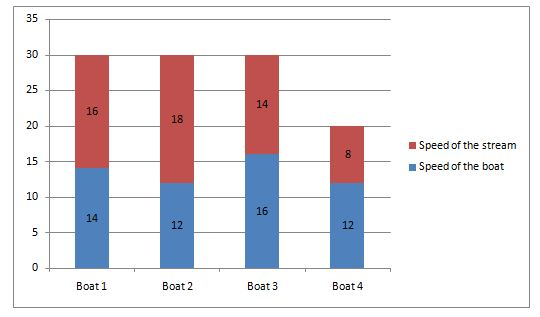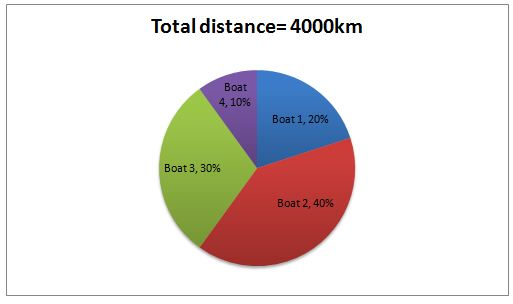# Quantitative Aptitude Questions (Data Interpretation) for SBI PO Mains 2018 Day-149

Dear Readers, SBI is conducting Online Examination for the recruitment of probationary officer. To enrich your preparation here we have providing new series of Data Interpretation – Quantitative Aptitude Questions. Candidates those who are appearing in SBI PO Mains Exams can practice these Quantitative Aptitude average questions daily and make your preparation effective.

Click “Start Quiz” to attend these Questions and view Solutions

Directions (1-5): Study the following table and answer the given questions.

 Country Name Total No. of Matches Played No. of Matches Won No. of Matches Lost No. of Matches Draw London – – 54 16 India – 120 50 – Japan 170 110 – – Canada 184 – 56 – Australia – 100 – –

1) How many countries has won more than 115 matches, if the total number of matches won by all the country  is 530 and total number of matches played by London is twice as match won by Australia?
a) 2

b) 4

c) 5

d) 3

e) 1

2) What is the number of matches draw by Australia, if the ratio between matches won by India and London together to the total number of matches played by Canada and Australia is 4:5 and the ratio between number of matches played by London and Japan together to number of matches lost by Canada and Australia is 5:3, if the number of matches lost by Australia is twice the number of matches lost by India?

a) 16

b) 45

c) 42

d) 23

e) 11

3) What is the total number of matches played by India, if the number of matches draw by India is 20% of the matches won by India?
a)  125

b) 197

c) 113

d) 125

e) 194

4) If Japan didn’t lose any match, then what is the difference between the percentage of matches drawn by Japan and percentage of matches lost by Canada?
a) 5%

b) 8%

c) 6%

d) 4%

e) 2%

5) If none of the match was drawn by any of the country, then what will be the ratio between matches played by India, Japan and Canada to number of matches lost by India, Japan and London?

a) 267: 67

b) 219: 67

c) 131:41

d) 161: 69

e) 165: 66

Directions (6-10): Study the following graphs and answer the given questions.6) If the speed of the Boat 1 is increased by 35% then what is the time taken by the same boat to reach 232 km if it travels in Upstream?

a) 80 hours

b) 47 hours

c) 26 hours

d) 46 hours

e) 56 hours

7) What is the ratio of total time taken by Boat 3 to the time taken by Boat 4 to reach their respective destination if they travel in downstream?

a) 2:1

b) 1:4

c) 8:1

d) 2:3

e) 5:1

8) What is the difference of time taken by Boat 1 to travel 750 km in downstream and time taken by Boat 2 to travel 570 km in downstream?

a) 2 hours

b) 6 hours

c) 7 hours

d) 9 hours

e) 4 hours

9) If a person travels 5280 km using all the four boats in a Week in the same direction of stream, then by travelling in which boat takes more time to finish his journey?

a) Boat 3

b) Boat 1

c) Boat 4

d) Boat 2

e) Boat 1 and 3

10) The Boat 2 is travelling in Downstream. If the speed of the Boat 2 is increased by 25% and the speed of stream is decreased by 10%, then what is the difference between time taken by the Boat 2 to travel 960 km in original speed and increased speed?

a) 6 hours

b) 2 hours

c) 5 hours

d) 3 hours

e) 1 hour

Direction (1-5)

From the table we observe that,

Total no. of matches played by London = 2*100 = 200

Now, No. of matches won by London = 200- (54+16) =130

Total no. of matches won by Canada = 530- (130+100+120+110)

= 70

Thus London and India have scores more than 115 matches.

Let the number of matches won by London and number of matches played by Australia be x and y respectively.

Then, according to the question,

[(120+x)/ (184+y)]=4/5

5(120 +x) = 4(184+y)

600 + 5x = 736 + 4y

5x – 4y = 736 – 600

5x – 4y = 136 ………………….. (1)

Let the matches played by London be p.

Then, Matches lost by Australia = 2*50=100

Then, [(p+ 170)/ (56+100)]= 5/3

After solving this we have p= 270

Thus x= 270-(54+16) = 200

Using this in (1), we have y=216

Therefore, matches draw by Australia= 216- (100 +100) =16

Total number of matches drawn by India = (20/100)* 120 = 24

Total number of matches Played by India =120 + 50 +24 = 194

Total number of matches drawn by Japan= 170- (110+0) = 60

Then percentage of matches drawn by Japan = (60/170)* 100 =35 %

Similarly, percentage of matches lost by Canada = (56/184) * 100 = 30%

Required difference= 35%-30%=5%

From the table we observe that,

Total number of matches played by India =120+50 =170

Total number of matches played by Japan = 170

Total number of matches played by Canada = 184

Total number of matches lost by India =50

Total number of matches lost by Japan = 170 – 110 = 60

Total number of matches lost by London = 54

Required ratio= (170+170+184): (50+60+54)

= 524: 164

=131: 41

Direction (6-10)

The speed of the Boat 1=135*14/100=18.9 km/hr

We know that, Time=Distance/Speed

Then Required time= 232/ (18.9-16)

=80 hours

We know that, time= distance/speed

Now, Time taken by Boat 3 = (30*4000)/ (100*30)

= 40 hours

Similarly, Time taken by Boat 4= (10*4000)/ (100*20)

= 20 hours

Required ratio= 40:20

= 2:1

Time taken by Boat 1=750/ (14+16) =25 hours

And Time taken by Boat 2=570/ (12+18) =19 hours

Required difference=25-19=6 hours

Since the boat travels in the same direction of stream, it travels in downstream.

Time taken by the person if he travels in Boat 1= 5280/ (14+16)

=176 hours

Time taken by the person if he travels in Boat 2=5280/ (12+18)

=176 hours

Time taken by the person if he travels in Boat 3 boat=5280/ (16+14)

= 176 hours

Time taken by the person if he travels in Boat 4= 5280/ (12+8)

= 264 hours.

Thus the person has to spend more time by travelling in the Boat 4.

Original Time taken by the boat= 960/ (12+18) =32 hours

Now, the new speed of the Boat 2= 125*12/100=15 km/hr

And the speed of stream = 18*90/100=16.2 km/hr

Time taken by the Boat 2=960/(15+16.2)

= 960/31.2

= 31 hours (approximately)

Required difference= 32-31= 1 hr

Daily Practice Test Schedule | Good Luck

 Topic Daily Publishing Time Daily News Papers & Editorials 8.00 AM Current Affairs Quiz 9.00 AM Quantitative Aptitude “20-20” 11.00 AM Vocabulary (Based on The Hindu) 12.00 PM General Awareness “20-20” 1.00 PM English Language “20-20” 2.00 PM Reasoning Puzzles & Seating 4.00 PM Daily Current Affairs Updates 5.00 PM Data Interpretation / Application Sums (Topic Wise) 6.00 PM Reasoning Ability “20-20” 7.00 PM English Language (New Pattern Questions) 8.00 PM